# Calculating the Length of Time (n)

There are occasions when we need to determine the length of time (n) in a Present Value (PV) calculation. To do this, we need to know the three other components in the PV calculation: present value amount, future cash amount (FV), and the interest rate used for discounting the future cash amount (i).

### Exercise #5

What is the length of time involved if a future amount of \$5,000 has a present value of \$1,000, and the time value of money is 8% compounded annually?

The following timeline depicts the information we know, along with the unknown component (n):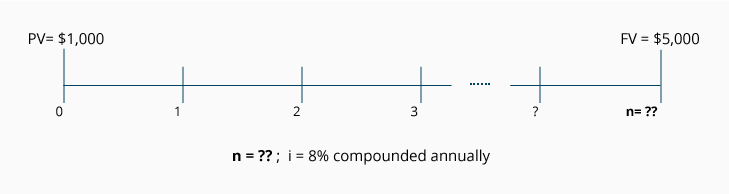Calculation Using the Present Value of 1 Table
As we had done in Part 3, we start with the PV formula: PV = FV x [ 1 ÷ (1 + i)n ]. We then substitute the PV of 1 factor for the expression "[ 1 ÷ (1 + i)n ]". Our equation now reads: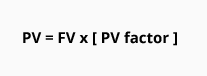From the information we've been given, we know that the future value is \$5,000, the present value is \$1,000, and the annual interest rate is 8% compounded annually. Let's plug those numbers into our equation to solve for (n), the number of annual time periods: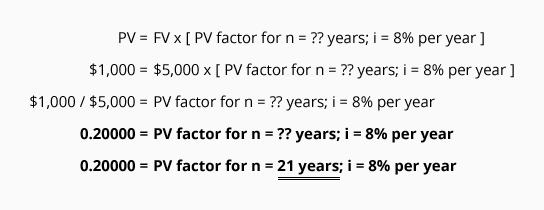Our equation tells us that the PV of 1 factor is 0.20040. Since the rate for discounting is 8% compounded annually, we look at the PV of 1 Table in the 8% column and find the amount closest to 0.200; this would be 0.199. We see it is located in the row where n = 21. This tells us that the missing component, length of time, is approximately 21 years.

### Exercise #6

What is the length of time involved if a future amount of \$10,000 has a present value of \$3,000, and the time value of money is 10% per year compounded semiannually?

The following timeline depicts the information we know, along with the unknown component (n):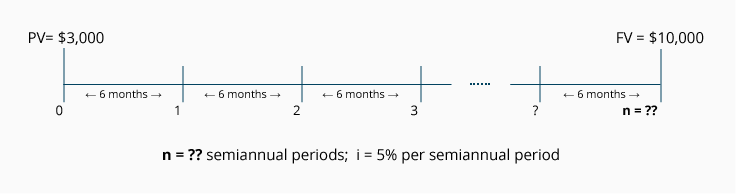Calculation Using a PV of 1 Table
As mentioned previously, if you are furnished with a table containing present value factors, the formula PV of 1 = FV times [ 1 ÷ (1 + i)n ], can be restated to:From the information we've been given, we know that the future value is \$10,000 and the present value is \$3,000. The annual interest rate is 10% compounded semiannually, which we can restate as 5% per semiannual period. When we plug in the information we know, we can solve for (n), the number of semiannual periods: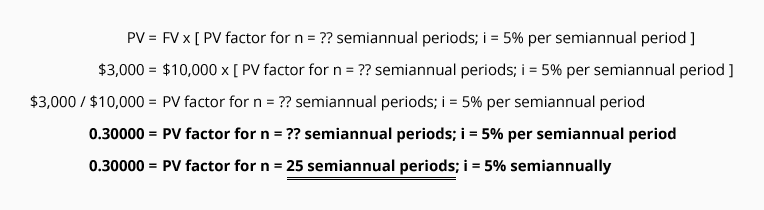Our equation tells us that the PV factor is 0.30000. Since the rate for discounting is 5% per semiannual period, we look at the PV of 1 Table in the 5% column and find the amount closest to 0.300; this would be 0.295. We see it is located in the row where n = 25. This tells us that the missing component, length of time, is approximately 25 semiannual periods (about 12.5 years).

### Exercise #7

What is the length of time involved if a future amount of \$100,000 has a present value of \$75,000, and the time value of money is 12% per year compounded monthly?

The following timeline depicts the information we know, along with the unknown component (n):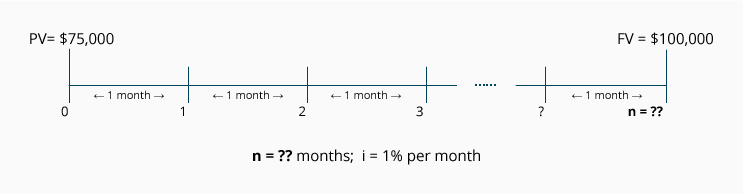Calculation Using a PV of 1 Table
From the information we've been given, we know that the future value is \$100,000 and the present value is \$75,000. The annual interest rate is 12% compounded monthly, which we can restate as 1% per month. Let's plug in the information we know and solve for (n):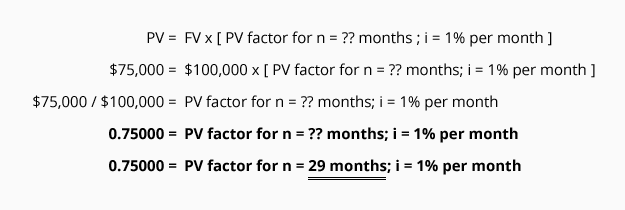Our equation tells us that the PV factor is 0.750. Since the rate for discounting is 1% per month, we look at the PV of 1 Table in the 1% column and find the amount closest to 0.750; this amount would be 0.749. We see it is located in the row where n = 29. This tells us that the missing component, length of time, is approximately 29 months (about 2.4 years).